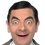Hi Brilliant,

I've been self-learning from a physics textbook called Fundamentals of Physics. I'm doing a bit of everything, but there's this optics problem that I just don't understand. Here's the problem (paraphrased).

A swimming pool has a depth of 200 cm. A small light bulb is suspended 250 cm above the surface of the water. The bottom of the pool is a large plane mirror. At what depth below the mirror is the image of the light bulb? (Water has an index of refraction of 1.33.)

Apparently, the answer is 351 cm, but I keep on getting 1.33*(250 cm)+200 cm = 532.5 cm. I assumed a light ray was coming off the light bulb at a small angle theta to the vertical. It would then refract to get an even smaller angle phi in the water. The light ray in the water would then reflect, and you would see the image on the extension of the reflection. However, the image wouldn't be shifted to the left or right, so you would also see the image on a straight axis below the light bulb. I then used the small angle approximations (sinx=tanx=x) and a bit of geometry to get my answer.

Can anyone explain where I went wrong? Or is there a typo in the textbook?Note by Steven Mai
4 years, 10 months ago

This discussion board is a place to discuss our Daily Challenges and the math and science related to those challenges. Explanations are more than just a solution — they should explain the steps and thinking strategies that you used to obtain the solution. Comments should further the discussion of math and science.

When posting on Brilliant:

• Use the emojis to react to an explanation, whether you're congratulating a job well done , or just really confused .
• Ask specific questions about the challenge or the steps in somebody's explanation. Well-posed questions can add a lot to the discussion, but posting "I don't understand!" doesn't help anyone.
• Try to contribute something new to the discussion, whether it is an extension, generalization or other idea related to the challenge.

MarkdownAppears as
*italics* or _italics_ italics
**bold** or __bold__ bold
- bulleted- list
• bulleted
• list
1. numbered2. list
1. numbered
2. list
Note: you must add a full line of space before and after lists for them to show up correctly
paragraph 1paragraph 2

paragraph 1

paragraph 2

[example link](https://brilliant.org)example link
> This is a quote
This is a quote
    # I indented these lines
# 4 spaces, and now they show
# up as a code block.

print "hello world"
# I indented these lines
# 4 spaces, and now they show
# up as a code block.

print "hello world"
MathAppears as
Remember to wrap math in $$ ... $$ or $ ... $ to ensure proper formatting.
2 \times 3 $2 \times 3$
2^{34} $2^{34}$
a_{i-1} $a_{i-1}$
\frac{2}{3} $\frac{2}{3}$
\sqrt{2} $\sqrt{2}$
\sum_{i=1}^3 $\sum_{i=1}^3$
\sin \theta $\sin \theta$
\boxed{123} $\boxed{123}$

Sort by:

I think a typo

- 4 years, 4 months ago